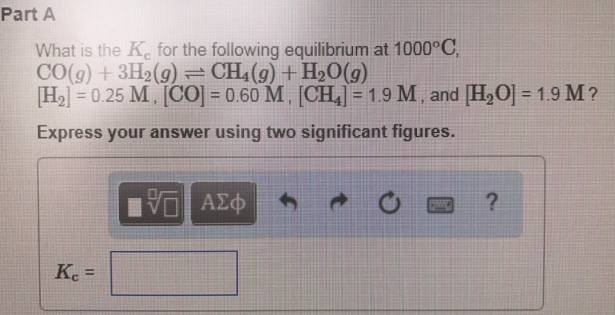# Problem: What is the Kc for the following equilibrium at 1000°C, CO (g) + 3H2 (g) ⇌ CH4 (g) + H2O (g) [H2] = 0.25 M, [CO] = 0.60 M, [CH 4] = 1.9 M, and [H2O] = 1.9 M? Express your answer using two significant figures.

###### FREE Expert Solution
86% (395 ratings)###### Problem Details

What is the Kc for the following equilibrium at 1000°C,

CO (g) + 3H2 (g) ⇌ CH4 (g) + H2O (g)

[H2] = 0.25 M, [CO] = 0.60 M, [CH 4] = 1.9 M, and [H2O] = 1.9 M?